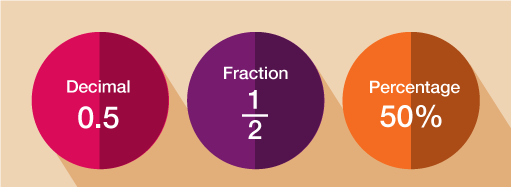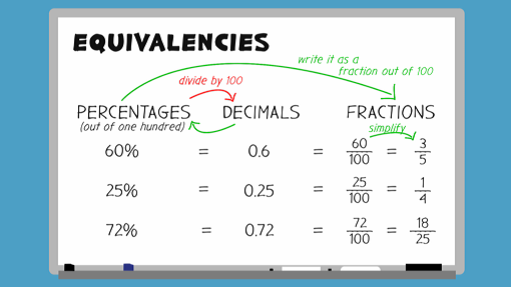Science, Maths & Technology

### Become an OU studentEveryday maths 2

Start this free course now. Just create an account and sign in. Enrol and complete the course for a free statement of participation or digital badge if available.

# 6 Fractions, decimals and percentages

You have already worked with decimals in this course and many times throughout your life. Every time you calculate something to do with money, you are using decimal numbers. You have also learned how to round a number to a given number of decimal places.Figure _unit2.6.1 Figure 8 Equivalent decimals, fractions and percentages

Since fractions, decimals and percentages are all just different ways of representing the same thing, we can convert between them in order to compare. Take a look at the video below to see how to convert fractions, decimals and percentages.Interactive feature not available in single page view (see it in standard view).

## Activity _unit2.6.1 Activity 12: Matching fractions, decimals and percentages

Choose the correct fraction for each percentage and decimal.

Using the following two lists, match each numbered item with the correct letter.

• a.      35% = 0.35 =

• b.      62.5% = 0.625 =

• c.      50% = 0.5 =

• d.      40% = 0.4 =